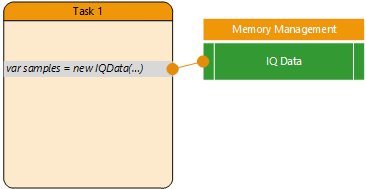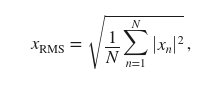## IQdata (IQ samples)

The IQ Data object handles many operations related to multi-channel complex samples.

Warning

The channel numbering starts from 0.
For example, an IQData object where getChannelCount() == 2 :

• the fist channel has index 0
• the second channel has index 1.
``````class IQData {
constructor IQData( [string name] );

dump() ; // prints details on the std out
forceFloat(); // make sure internal data is in float format and not in original format
number getChannelCount() ; // number of channels in the data , by default 1

// load IQ samples from an existing file, supported formats : CS16, CF32, WAV
// Save IQ Data to a file, supported formats : CS16, CF32, WAV
boolean saveToFile( string filename  , [option:channel]);
// append IQ Data to a file, supported formats : CS16, CF32 (no WAV)
bool appendToFile( string filename  , [option:channel]);

getSamples( int start, array ,[option:channel]); - where array : a = new Float32Array(2*size)
setSamples( int start, array ,[option:channel]);

Samples getReal([option:channel])  ; // returns real part
Samples getImag([option:channel])  ; // returns imag part

freqShift( number freqHz );

number getLength() ; // length in samples
setLength( number of samples , number_of_channels) ; // truncate or extend

number getSampleRate();
setSampleRate( number );  // change the samplerate

number getCenterFrequency();
setCenterFrequency( number );

number getTimestamp(); // returns the timestamp for the first sample of this block (milliseconds since 01/01/1970)
number getDuration() ; // returns the duration in seconds ( number of samples / sample rate)
Object getGPS() ; // returns coordinates associated to this IQ Data block

number rms( [option:channel] );

object getAC( length , [option:channel]) ; // returns autocorrelation object
object getPowerSpectrum( spectrum_width , [option:channel]);
bool   CSVSpectrum( spectrum_width, filename, [option:channel]);

IQData part( number sart, number samples , [option:channel]); // extract a subpart of the IQ
bool append( IQData );

readFromQueue( IQQueue object ) ; // replaces current samples with what is in the queue - waits (locks task)

FloatData getReal() ; // returns the real part in a FloatData object
FloatData getImag() ; // returns the imaginary part in a FloatData object

// Attribute management
bool setAttribute( JSONObjet ); // Add an attribute JSON object to the IQ Data
JSONObjet getAttribute();       // retrieve attribute object

Object toJSON( start, count ) ; // convert to a Javascript representation the internal data, from start to start+count

}
``````

Memory management:Samples are stored in the host computer RAM. Only a reference (a memory pointer) to the data is stored in the task memory.
The Virtual Machine tries to keep the data in the original format as long as possible and convert it to float only when required.
Typically :

• A capture from a radio device will generate integer-type data, the IQData pointer will reference integer data,
• As soon as you want to access the data as floats (typically call a getPowerSpectrum() function for example), data is first converted to floating point.

Note: Default samplerate for IQ object is 1Msps. Use `IQ.setSampleRate(SR_Hz)` command to set samplerate for a given IQ object.

• Example :
``````// Define and load IQ object
var o = new IQData('');
exit();
}
// Set frequency and samplerate
o.setCenterFrequency( 100 );
o.setSampleRate(8e6);

print('we have '+ o.getLength() + ' samples recorded at '+ o.getCenterFrequency() + ' MHz, Bandwidth : ' + o.getSampleRate() / 1e3 + ' kHz');
print('file length:' + o.getDuration() + ' secs.');

// Extract 1024 sample from offset 10 ,as array (1024 Isamples and 1024 Qsamples)
var rawIQ = new Float32Array(1024*2);
var n = o.getSamples( 10, rawIQ );
print('extracted ' + n + ' IQ Pairs');
We have loaded ' + satlist.length + ' sat definitions.');
``````

### .dump

Display details on IQ object (in the VM console).

``````var o = new IQData('');
exit();
}
o.dump();
``````

### .getChannelCount

Returns the number of channels contained in the IQData internal samples. For example, you may collect the two RF inputs from a Nuand BladeRF board. In this case the IQ data would contain two channels.

``````var rx = ...
var IQ = rx.CaptureAllChannels();
var channels = IQ.getChannelCount();
``````

### .getLength

Returns the number of samples stored internally.

• Example
``````var o = new IQData('');
print('We have loaded ' + o.getLength() + ' samples');
``````

### .setLength

Define length (samples number) of an IQ object. If the object is empty, the number of channels can be specified with the optional second argument

``````     setLength( number of samples [,number_of_channels] ) ;
``````

### .getSampleRate

Returns samplerate (Hz) of an IQ object

:!: If undefined, default samplerate is 1Msps

`````` .getSampleRate();
``````

### .getCenterFrequency

• Get center frequency of IQ object, value returned in MHz.
``````.getCenterFrequency();
``````

### .setSampleRate

Define a samplerate for an IQ object

``````.setSampleRate(SR_Hz);
``````
• Example
``````var o = new IQData('');
o.setSampleRate(8e6);
``````

### .setCenterFrequency

Set the senter frequency (MHz) of an IQ object

``````.setCenterFrequency(Freq_MHz);
``````
``````var o = new IQData('/tmp/test.cf32');
o.setCenterFrequency(98);
var x = o.getCenterFrequency();
print(x);
``````

### .getDuration

Get IQ object duration, based on (number of samples)*(1/sample_rate)

Warning

You have to define samplerate ''.setSampleRate(SR_Hz)'' first.

• Example:

``````var o = new IQData('/tmp/test.cf32');
o.setSampleRate(8e6);
print('Size:' + o.getLength() + "  - SR: "  o.getSampleRate() + "  - duration: " o.getDuration() + ' secs.');
``````

### .getTimestamp

Get UNIX timestamp of an IQ block (milliseconds since 01/01/1970)

``````var x = new IQData('x');
var timestamp = x.getTimeStamp();
``````

### .rms

Returns the log RMS power of the IQ data.

• The returned value is : 20*log10( rms_power ),
• The rms is computed as :using all the samples from the IQData object

``````var o = new IQData('');
exit();
}
``````

### .saveToFile

Save IQ object to file. Exisiting file is replaced (use .appendToFile() to add samples to exisiting file )
In case of a multiple channel IQData, the channel to be saved has to be specified (otherwise, only channel 0 will be stored).

Following formats are handled by recognizing their extension name.

-.CF32 ou .FC32 ou .IQ32 : Complex float single precision (8 bytes by sample, 4 for I, 4 for Q)
-.CS16 : Complex signed integer : 16 bits
-.CS8 : Complex signed integer: 8 bits
-.WAV : format Audio stereo
-.SDR : Custom format mixing metadata and IQ values, support multichannels datas

``````.saveToFile(filename, [option:channel]);
``````
• Example:
``````radio.setRxCenterFreq( 466 );
var IQ = radio.Capture( 10000 );
IQ.dump();
IQ.saveToFile('capture.cf32') ;
``````

### .appendToFile

Similar to .saveToFile() except appends to data to file if exisiting.
In case of a multiple channel IQData, the channel to be saved has to be specified (otherwise, only channel 0 will be stored).

Following formats are handled by recognizing their extension name.

-.CF32 ou .FC32 ou .IQ32 : Complex float single precision (8 bytes by sample, 4 for I, 4 for Q)
-.CS16 : Complex signed integer : 16 bits
-.CS8 : Complex signed integer: 8 bits
-.SDR : Custom format mixing metadata and IQ values, support multichannels datas

`Warning: WAV file format is not supported in current versions.`

### .getPowerSpectrum

This function computes the average power spectrum using the Fast Fourrier Transform. An object is returned :

``````{
"datatype": "power_spectrum",
"fft_size": your_fft_size,
"timestamp": number, /* number of milliseconds since 01/01/1970 */
"position" : {
"gps_fix": boolean,
"latitude_N": number,
"longitude_E": number,
"altitude" : number
}
"peaks" : [ array of peaks, see below ],
"spectrum": []
}
``````
``````var IQ = radio.Capture( 10000 );
var spectrum = IQ.getPowerSpectrum( fft ) ;
peaks = spectrum.peaks ;
print('Peaks object:' + JSON.stringify( peaks ));
``````
``````[
{"frequency":0, "value":-99.626,"index":256},
{"frequency":-0.038, "value":-114.254, "index":158},
{"frequency":-0.047, "value":-114.162, "index":133},
...
]
``````

### .CSVSpectrum

Compute the average power spectrum using the Fast Fourrier Transform and export to CSV file.

• If the file does not exist, a header line is added first,
• In case the specified file already exists, the values are added as a new line.
``````bool CSVSpectrum( fft_size, filename, [option:channel]) ;
``````

Parameters:

• fft_size number, size of the Fast Fourrier Transform to be used.
• filename string, the file name.

This function returns true if the file has been written, false otherwise.

Example :

``````var rx = Soapy.makeDevice( {'query' : 'driver=rtlsdr' });
rx.setRxSampleRate( 2e6 );
rx.setRxCenterFreq( 105.5 );
for( var i=0 ; i < 100 ; i++ ) {
var samples = rx.Capture( 2e6 ) ;
samples.CSVSpectrum( 1024, 'test.csv');
}
``````

### .getAC

``````.getAC( length , [option:channel]) ;
``````

This function computes the autocorrelation normalized coefficients for the IQ data using the given length.
- returns autocorrelation object

### .getSamples

Extract samples from IQ object, from position 'start'
Array format : a = new Float32Array(2*size)

``````getSamples(start, array)
``````
• Example
``````var o = new IQData('');
print(o.getLength() + ' samples');
var rawIQ = new Float32Array(1024*2);
var n = o.getSamples( 10, rawIQ );
print('extracted ' + n + ' IQ Pairs');
``````

### .part

Extracts a subset of samples from an IQ object. For multi-channel IQ objects, an optional integer has to be provided to set from which channel the data has to be extracted.
First channel is at index 0.

``````.part(offset_start,size, [option int channel]);
``````
• Example
``````var o = new IQData('');
// get 1024 complex from o, starting at index 1024
var subdata = o.part( 1024, 1024) ;
``````

### .append

Append IQ samples to existing IQ object

``````.append(IQ_data);
``````
• Example :
``````var IQ = DSP.tone( 440, 1000, 12500 );
IQ.append( DSP.tone( 880, 1000, 12500) );
IQ.dump();
IQ.saveToFile('/save/tone.cf32');
``````

### .freqShift

Shift center frequency using offset : freq_Hz. The object sample rate is used to compute the Local Oscillator value to be applied. Make sure the object has a valid samplerate (>0) before call

``````.freqShift(freq_Hz);
``````
``````// load the searched signal
var IQ = new IQData('');
// shift the searched signal
IQ.freqShift( -300 );
IQ.dump();
``````

### .setAttribute

Attach a JSON object to the IQ Data. This will be forwarded to queues.

Example :

``````var IQ = DSP.tone( 440, 1000, 1000 );

var x = { 'field_a' : 4, 'field_b' : 12 } ;
IQ.setAttribute( x );
``````

### .getAttribute

Extracts attribute associated to IQ datas.

Example :

``````var q = Queues.create( 'queue');
// Get a block
var IQ = q.dequeue(true); // blocking mode, we wait to have something
var x = IQ.getAttribute();
``````
Last update: March 15, 2023